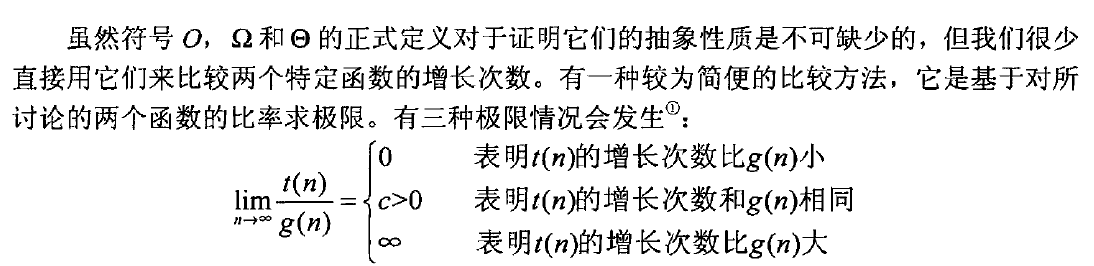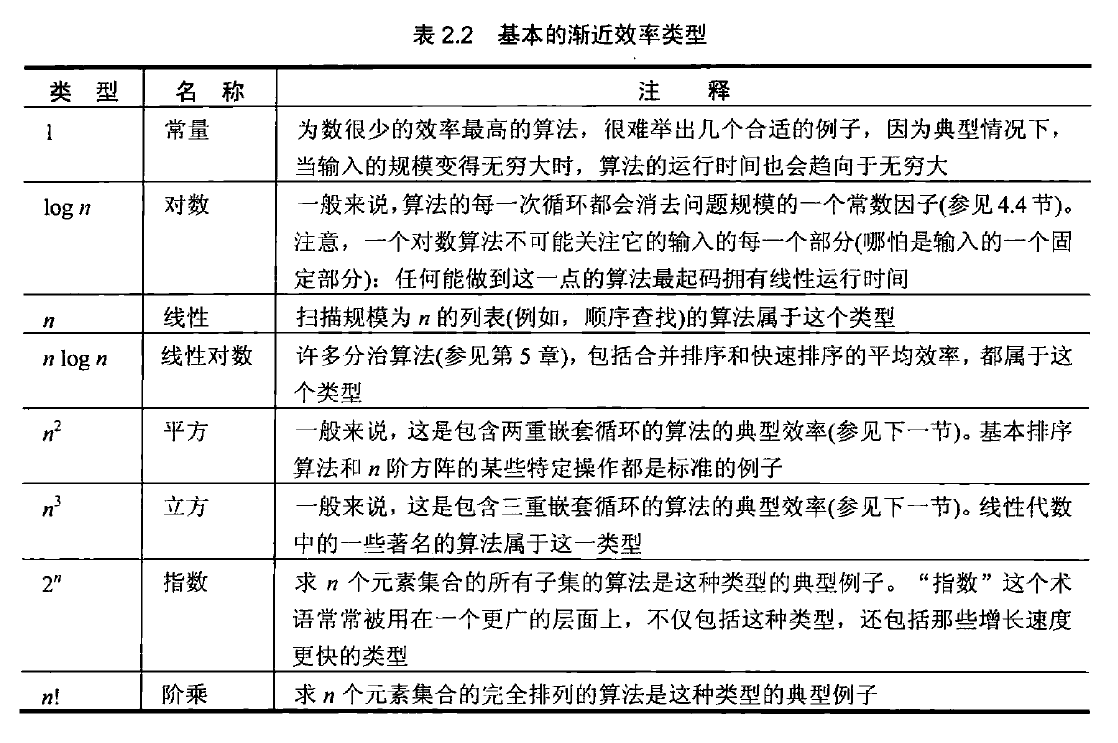# 算法笔记-算法效率分析基础

Posted by MaggicQ on July 1, 2017

# 算法效率分析

• 最优效率
• 最差效率
• 平均效率，必要性：有很多重要的算法的平均效率比他们的最差效率要好很多。

## 渐进符号

$O(g(n))$ 是增长次数小于等于 $g(n)$ (及其常数倍， $n$ 趋向于无穷大)的函数集合。举几个例子：$n \in O(n^2) ,100n + 5 \in O(n^2), n^2-2n \in O(n^2)$

$\Omega(g(n))$ 代表增长次数大于等于 $g(n)$ 的函数集合。$\Theta(g(n))$ 是增长次数等于 $g(n)$ 的函数集合。

### 特性：### 利用极限比较增长次数### 基本的渐进效率类型### 分析非递归算法效率的通用方案

• 决定用哪个参数表示输入规模
• 找出算法的基本操作（一般位于算法的最内层循环中）
• 检查基本操作的执行次数是否只依赖于输入规模，如果它还依赖于一些其他的特性，则最差效率、平均效率以及最优效率需要分别研究
• 建立一个算法基本操作执行次数的求和表达式
• 利用求和运算的标准公式和法则来建立一个操作次数的闭合公式，或者至少确定它的增长次数

### 分析递归算法时间效率的通用方案

• 决定用哪个参数作为输入规模的度量标准
• 找出算法的基本操作
• 检查基本操作的执行次数是否只依赖于输入规模，如果它还依赖于一些其他的特性，则最差效率、平均效率以及最优效率需要分别研究
• 对于算法基本操作的执行次数，建立一个递推关系以及相应的初始条件
• 解这个递推式，或者至少确定它的解的增长次数